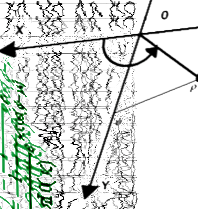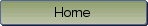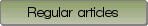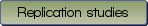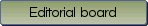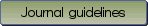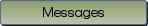A review of effect sizes and their confidence intervals, Part I: The Cohen's d family

Bibliographic information: BibTEX format RIS format XML format APA style
Cited references information: BibTEX format APA style

Goulet-Pelletier, Jean-Christophe , Cousineau, Denis
242-265
Keywords: Effect size , standard error , confidence intervals , Cohen's $d$ , noncentral $t$-distribution
(no sample data)   (Appendix)

There has been an erratum in volume 15(1), p. 54

Effect sizes and confidence intervals are important statistics to assess the magnitude and the precision of an effect. The various standardized effect sizes can be grouped in three categories depending on the experimental design: measures of the difference between two means (the $d$ family), measures of strength of association (e.$g$., $r$, $R^2$, $\eta ^2$, $\omega ^2$), and risk estimates (e.g., odds ratio, relative risk, phi; Kirk, 1996). Part I of this study reviews the $d$ family, with a special focus on Cohen's $d$ and Hedges' $g$ for two-independent groups and two-repeated measures (or paired samples) designs. The present paper answers questions concerning the $d$ family via Monte Carlo simulations. First, four different denominators are often proposed to standardize the mean difference in a repeated measures design. Which one should be used? Second, the literature proposes several approximations to estimate the standard error. Which one most closely estimates the true standard deviation of the distribution? Lastly, central and noncentral methods have been proposed to construct a confidence interval around $d$. Which method leads to more precise coverage, and how to calculate it? Results suggest that the best way to standardize the effect in both designs is by using the pooled standard deviation in conjunction with a correction factor to unbias $d$. Likewise, the best standard error approximation is given by substituting the gamma function from the true formula by its approximation. Lastly, results from the confidence interval simulations show that, under the normality assumption, the noncentral method is always superior, especially with small sample sizes. However, the central method is equivalent to the noncentral method when $n$ is greater than 20 in each group for a between-group design and when $n$ is greater than 24 pairs of observations for a repeated measures design. A practical guide to apply the findings of this study can be found after the general discussion.

Be informed of the upcoming issues with RSS feed:RSS